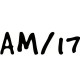# CBSE Class 12 Maths Notes Integrals & FormulasWritten by

Integrals & Formulas is part of Class 12 Maths Notes for Quick Revision. Here we have given Class 12 Maths Notes Integrals & Formulas.Integrals

Integration is the inverse process of differentiation. In the differential calculus, we are given a function and we have to find the derivative or differential of this function, but in the integral calculus, we are to find a function whose differential is given. Thus, integration is a process which is the inverse of differentiation.
Then, ∫f(x) dx = F(x) + C, these integrals are called indefinite integrals or general integrals. C is an arbitrary constant by varying which one gets different anti-derivatives of the given function.
Note: Derivative of a function is unique but a function can have infinite anti-derivatives or integrals.

## Properties of Indefinite Integral

(i) ∫[f(x) + g(x)] dx = ∫f(x) dx + ∫g(x) dx
(ii) For any real number k, ∫k f(x) dx = k∫f(x)dx.
(iii) In general, if f1, f2,………, fn are functions and k1, k2,…, kn are real numbers, then
∫[k1f1(x) + k2 f2(x)+…+ knfn(x)] dx = k1 ∫f1(x) dx + k2 ∫ f2(x) dx+…+ kn ∫fn(x) dx

## Basic Formulae## Integration using Trigonometric Identities

When the integrand involves some trigonometric functions, we use the following identities to find the integral:

• 2 sin A . cos B = sin( A + B) + sin( A – B)
• 2 cos A . sin B = sin( A + B) – sin( A – B)
• 2 cos A . cos B = cos (A + B) + cos(A – B)
• 2 sin A . sin B = cos(A – B) – cos (A + B)
• 2 sin A cos A = sin 2A
• cos2 A – sin2 A = cos 2A
• sin2 A = ($\frac { 1-cos2A }{ 2 }$)
• sin2 A + cos2 A = 1
•${ sin }^{ 3 }A=\frac { 3sinA-sin3A }{ 4 }$
•${ cos }^{ 3 }A=\frac { 3cosA+cos3A }{ 4 }$

## Integration by Substitutions

Substitution method is used, when a suitable substitution of variable leads to simplification of integral.
If I = ∫f(x)dx, then by putting x = g(z), we get
I = ∫ f[g(z)] g'(z) dz
Note: Try to substitute the variable whose derivative is present in the original integral and final integral must be written in terms of the original variable of integration.

## Integration by Parts

For a given functions f(x) and q(x), we have
∫[f(x) q(x)] dx = f(x)∫g(x)dx – ∫{f'(x) ∫g(x)dx} dx
Here, we can choose the first function according to its position in ILATE, where
I = Inverse trigonometric function
L = Logarithmic function
A = Algebraic function
T = Trigonometric function
E = Exponential function
[the function which comes first in ILATE should taken as first junction and other as second function]

Note
(i) Keep in mind, ILATE is not a rule as all questions of integration by parts cannot be done by above method.
(ii) It is worth mentioning that integration by parts is not applicable to product of functions in all cases. For instance, the method does not work for ∫√x sinx dx. The reason is that there does not exist any function whose derivative is √x sinx.
(iii) Observe that while finding the integral of the second function, we did not add any constant of integration.

## Integration by Partial Fractions

A rational function is ratio of two polynomials of the form$\frac { p(x) }{ q(x) }$, where p(x) and q(x) are polynomials in x and q(x) ≠ 0. If degree of p(x) > degree of q(x), then we may divide p(x) by q(x) so that$\frac { p(x) }{ q(x) } =t(x)+\frac { { p }_{ 1 }(x) }{ q(x) }$, where t(x) is a polynomial in x which can be integrated easily and degree of p1(x) is less than the degree of q(x) .$\frac { { p }_{ 1 }(x) }{ q(x) }$ can be integrated by expressing$\frac { { p }_{ 1 }(x) }{ q(x) }$ as the sum of partial fractions of the following type:where x2 + bx + c cannot be factorised further.

Integrals of the typescan be transformed into standard form by expressingIntegrals of the typescan be transformed into standard form by expressing px + q = A$\frac { d }{ dx }$ (ax2 + bx + c) + B = A(2ax + b) + B, where A and B are determined by comparing coefficients on both sides.

We hope the given CBSE Class 12 Maths Notes Integrals will help you. If you have any query regarding NCERT Class 12 Maths Notes Integrals, drop a comment below and we will get back to you at the earliest.

## Class 12 Maths Notes

Relations and Functions

Inverse Trigonometric Functions

Matrices

Determinants

Continuity and Differentiability

Application of Derivatives

Integrals

Application of Integrals

Differential Equations

Vector Algebra

Three Dimensional Geometry

Linear Programming

Probability